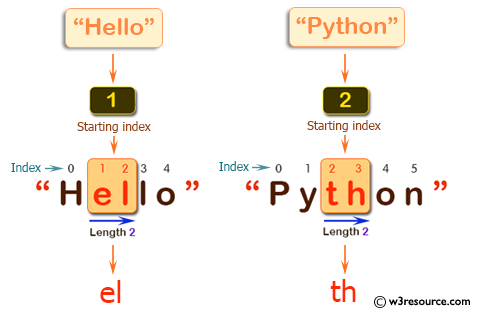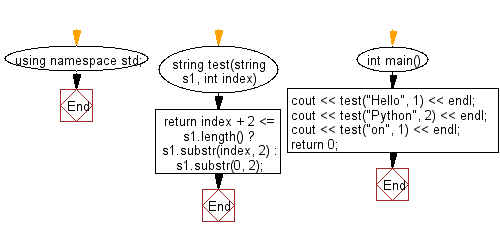﻿ C++ : A new string of length 2 starting at the given index# C++ Exercises: Create a new string of length 2 starting at the given index of a given string

## C++ Basic Algorithm: Exercise-69 with Solution

Write a C++ program to create a new string of length 2 starting at the given index of a given string.

Sample Solution:

C++ Code :

``````#include <iostream>
using namespace std;

string test(string s1, int index)
{
return index + 2 <= s1.length() ? s1.substr(index, 2) : s1.substr(0, 2);
}

int main()
{
cout << test("Hello", 1) << endl;
cout << test("Python", 2) << endl;
cout << test("on", 1) << endl;
return 0;
}
``````

Sample Output:

```el
th
on
```

Pictorial Presentation:Flowchart:C++ Code Editor: